Courses

# Short Questions With Answers - Theory Of Consumer Behaviour Commerce Notes | EduRev

## Commerce : Short Questions With Answers - Theory Of Consumer Behaviour Commerce Notes | EduRev

The document Short Questions With Answers - Theory Of Consumer Behaviour Commerce Notes | EduRev is a part of the Commerce Course Economics Class 11.
All you need of Commerce at this link: Commerce

Q.1. Define utility.
Ans.
Utility is the power to satisfy a want.

Q.2. Define Marginal Utility.
Ans.
Marginal Utility (MU) is the additional utility derived from consuming one more unit of a commodity.
MUn = TUn — TUn-1

Q.3. What is Total Utility?
Ans.
Total Utility is the total psychological satisfaction derived by a consumer from the consumption of total units of a good.
TUn= MU1 + MU2 + MU3 + MUn— ∑MU

Q.4. How are TU and MU related to each other?
Ans.
The relationship between TU and MU is as below
(i) Total Utility is the summation of Marginal Utility derived from each unit, that is, TU = ∑MU
(ii) TU increases so long as MU is positive.
(iii) When MU is zero, TU is maximum.
(iv) When MU is negative, TU starts decreasing.

Q.5. How is Marginal Utility derived from Total Utility?
Ans.
Marginal Utility can be derived from Total Utility as:
MUn = TUn - TUn-1

Q.6. State the shape of Marginal Utility curve?
Ans
. Marginal utility curve slopes downwards from left to right.

Q.7. What happens to TU when MU is negative?
Ans.
When MU is negative, TU is decreasing.

Q.8. What happens to TU when MU is positive?
Ans.
When MU is positive, TU is increasing.

Q.9. What happens to MU when TU is maximum?
Ans.
When TU is maximum, MU is zero.

Q.10. Is the Law of Diminishing Marginal Utility applicable in case of money?
Ans.
When worth of a rupee is more to the poor than to the rich, then the law of diminishing MU should be applicable in case of money.

Q. 11. Describe the Law of Diminishing Marginal Utility.
Ans.
The Law of Diminishing Marginal Utility states that the amount of good 2 that the consumer is willing to give up for an additional unit of good I diminishes as the amount of good I increases. When consumers continuously consume units of a good, their Total Utility increases, but it increases at a diminishing rate. This law can also be clarified with the help of the following table and diagram: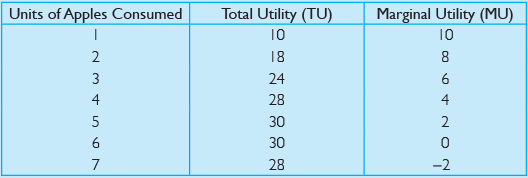This table and diagram highlight that as the consumption of units of apples increases, the utility derived from an additional unit decreases.
Total Utility is increasing at a diminishing rate. Total Utility is maximised when the consumer is consuming six units of apples because here the Marginal Utility is zero. This point is the point of maximum satisfaction. The Law of Diminishing Marginal Utility is based on the following assumptions:
(i) Interests and tastes of the consumer are unchanged.
(ii) Countable measurement of utility is possible.
(iii) Size, quality and shape of the every consumed unit remain the same.
(iv) Consumption of the commodity is continuous.
(v) Appropriate scale is used for consumption.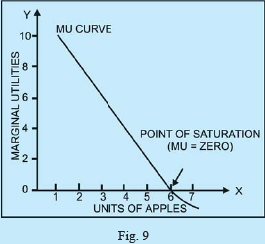Q.12. Explain the difference between cardinal utility and ordinal utility?
Ans.
Following are the points of different cardinal utility and ordinal utility: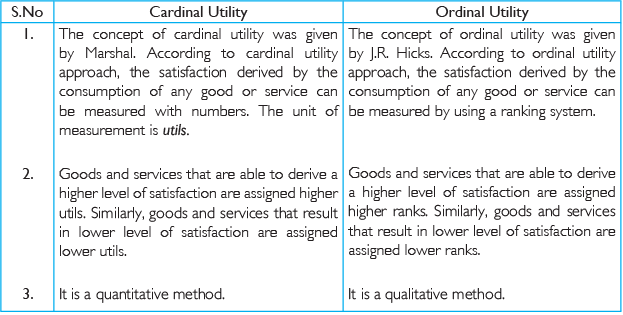Q.13. Describe the Law of Equi-Marginal Utility.
Ans.
When a consumer consumes only two commodities (X and Y), his or her equilibrium is determined in accordance with the law of equi-Marginal Utility. The consumer will allocate his or her money income between two goods in such a way that he or she gets equal Marginal Utility in terms of money from both the goods. Thus, the consumer will be in equilibrium when the ratio of the Marginal Utility of good X to price of X is equal to the ratio of the Marginal Utility of good Y to price of Y.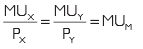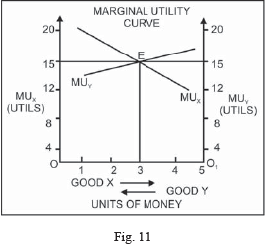In the diagram, when the consumer spends 3 units of money on good X and 2 units of money on good Y, he or she gets equal Marginal Utility of 16 units When Marginal Utilities are equal, Total Utility is maximised. The equilibrium point is shown by point Eon the diagram.

Q.14. What is budget set? Explain what can lead to change in budget set?
Ans.
The budget set includes all the possible bundles of two goods (commodities) that a consume- can afford to buy with his or her income at the prevailing market prices. The budget set depends on the income of the consumer and the prices of the goods and services. Accordingly, a budget set will change under the following conditions:
(i) Change in the money income of the consumer
(ii) Change in the price of any of the goods

Q.15. Define an indifference curve.
Ans.
An indifference curve is a locus of all the points representing combination of two goods among which the consumer is indifferent.

Q.16. Define indifference map.
Ans.
The indifference map is a graph which represents a group of indifference curves, each of them representing a given level of satisfaction.

Q.17. What does an IC show?
Ans.
An IC shows different combination of two commodities, which yield equal satisfaction to the consumer.

Q.18. What is meant by monotonic preference?
Ans.
Consumer preferences are said to be monotonic if he or she chooses a bundle, which gives more of either both the goods or at least one good without reducing the quantity of the other.

Q.19. What does the slope of IC indicate?
Ans.
The slope of the indifference curve indicates the rate at which the consumer is just willing to substitute one good for the other, maintaining the same level of satisfaction.

Q.20. What is Marginal Rate of Substitution?
Ans.
Marginal Rate of Substitution measures the rate at which the consumer is just willing to substitute one good for the other, maintaining the same level of satisfaction.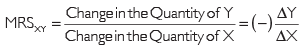Q.21. Describe the properties of indifference curve.
Ans.
Following are the properties of indifference curve
(i) Indifference curve slopes downwards from the left to right
(ii) Indifference curve is convex to the origin.
(iii) Two indifference curves never intersect each other.
(iv) Higher indifference curve represents a higher level of satisfaction.
(v) Indifference curves need not be parallel to each other.

Q.22. Describe the assumptions of indifference curve.
Ans.
Following are the main assumptions of indifference curve analysis
(i) The consumer is rational as he wants to maximise his satisfaction with his limited income.
(ii) The consumer consumes only two commodities.
(iii) There is ordinal measurement of utility, that is, utility is expressed in ranks
(iv) There is diminishing Marginal Rate of Substitution.
(v) There is non-satiety of the consumer.
(vi) The consumer is consistent and transitive in selection.

Q. 23 Define an indifference curve. Explain why an indifference curve is downward sloping from left to right?
Ans.
An indifference curve is a locus of all the points re-presenting combination of two goods among which the consumer is indifferent An indifference curve has a negative slope, that is, it slopes down-wards from left to right A consumer is confronted with various combinations of two goods, say, X and Y. Each combination gives him the same level of total satisfaction. If the total satisfaction is to remain the same, the consumer must part with diminishing units of Y as he or she gets an increasing stock of X. The loss of satisfaction to the consumer on account of the downwards movement is due to the gain through the rightward movement.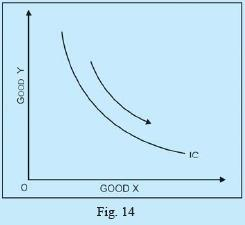Offer running on EduRev: Apply code STAYHOME200 to get INR 200 off on our premium plan EduRev Infinity!

## Economics Class 11

212 videos|194 docs|48 tests

,

,

,

,

,

,

,

,

,

,

,

,

,

,

,

,

,

,

,

,

,

;